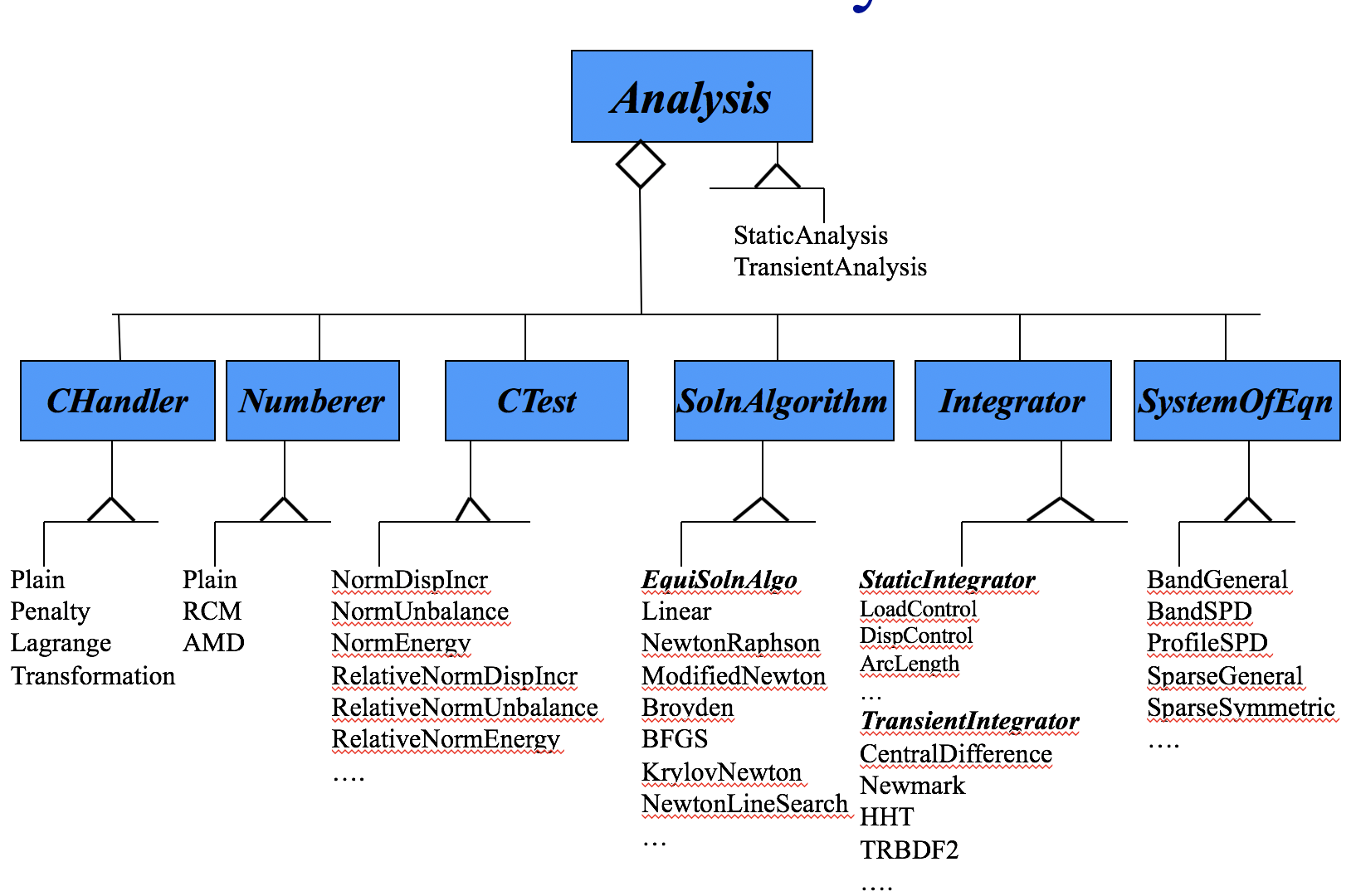# 3.2. Analysis Commands¶

In OpenSees, an analysis is an object which is composed by the aggregation of component objects. It is the component objects which define the type of analysis that is performed on the model. The component classes, as shown in the figure below, consist of the following:

1. Constraint Handler – determines how the constraint equations are enforced in the analysis – how it handles the boundary conditions/imposed displacements

2. DOF Numberer – determines the mapping between equation numbers in the system of equation and the degrees-of-freedom at the nodes

3. SystemOfEqn & Solver – it specifies how to store and solve the system of equations $$Ax=b$$

4. Convergence Test – determines when convergence has been achieved.

5. Solution Algorithm – determines the sequence of steps taken to solve the non-linear equation at the current time step

6. Integrator – determines the equations to solve, the predictive step, and how to update the response at the nodes given the solution to $$Ax=b$$Fig. 3.2.1 OpenSees Analysis